Chapter12 Electrostatic Phenomena Electric Forces and Electric Fields

• Slides: 14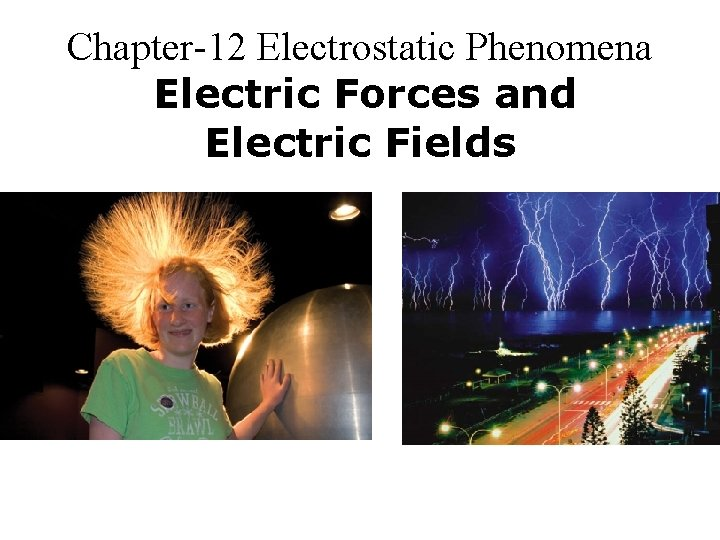Chapter-12 Electrostatic Phenomena Electric Forces and Electric Fields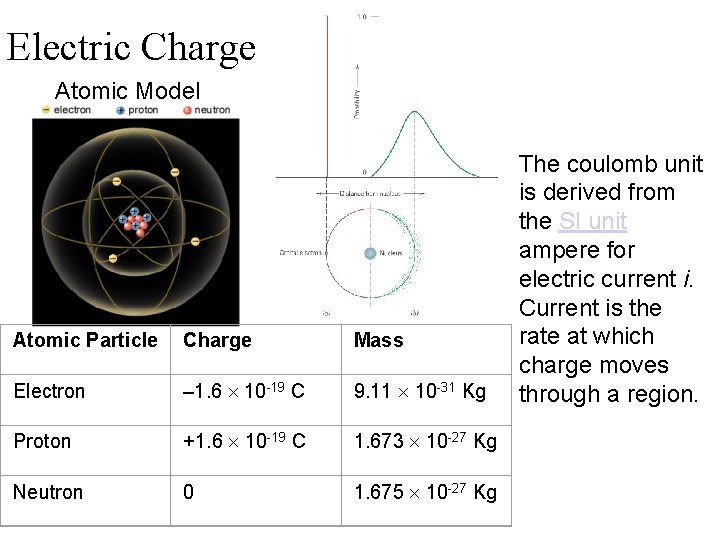Electric Charge Atomic Model Atomic Particle Charge Mass Electron – 1. 6 10 -19 C 9. 11 10 -31 Kg Proton +1. 6 10 -19 C 1. 673 10 -27 Kg Neutron 0 1. 675 10 -27 Kg The coulomb unit is derived from the SI unit ampere for electric current i. Current is the rate at which charge moves through a region.Charging Ebonite Rod & Fur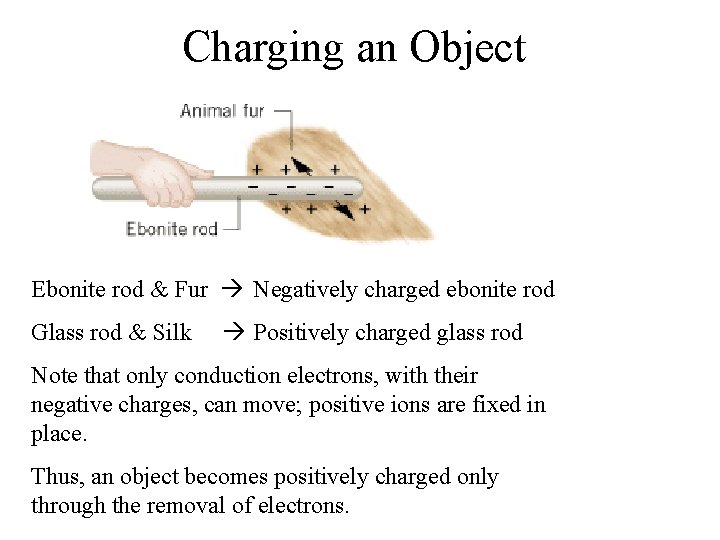Charging an Object Ebonite rod & Fur Negatively charged ebonite rod Glass rod & Silk Positively charged glass rod Note that only conduction electrons, with their negative charges, can move; positive ions are fixed in place. Thus, an object becomes positively charged only through the removal of electrons.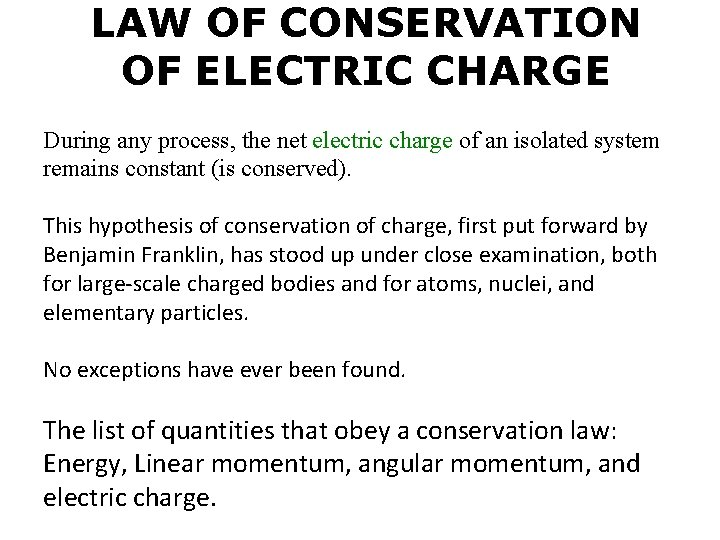LAW OF CONSERVATION OF ELECTRIC CHARGE During any process, the net electric charge of an isolated system remains constant (is conserved). This hypothesis of conservation of charge, first put forward by Benjamin Franklin, has stood up under close examination, both for large-scale charged bodies and for atoms, nuclei, and elementary particles. No exceptions have ever been found. The list of quantities that obey a conservation law: Energy, Linear momentum, angular momentum, and electric charge.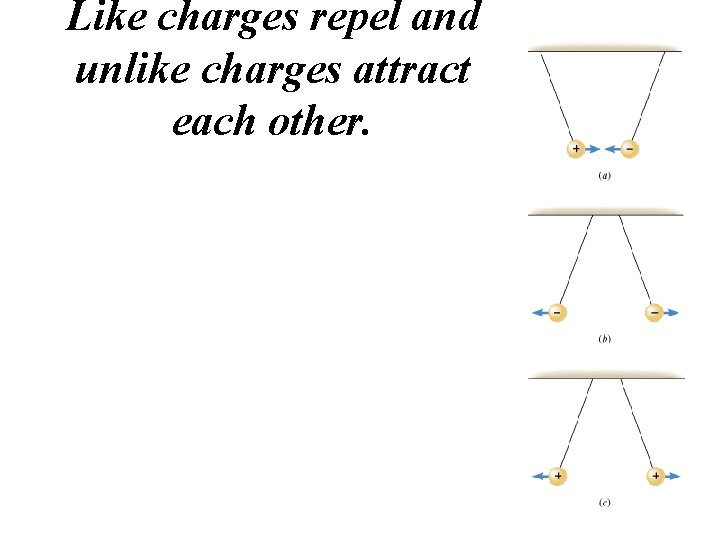Like charges repel and unlike charges attract each other.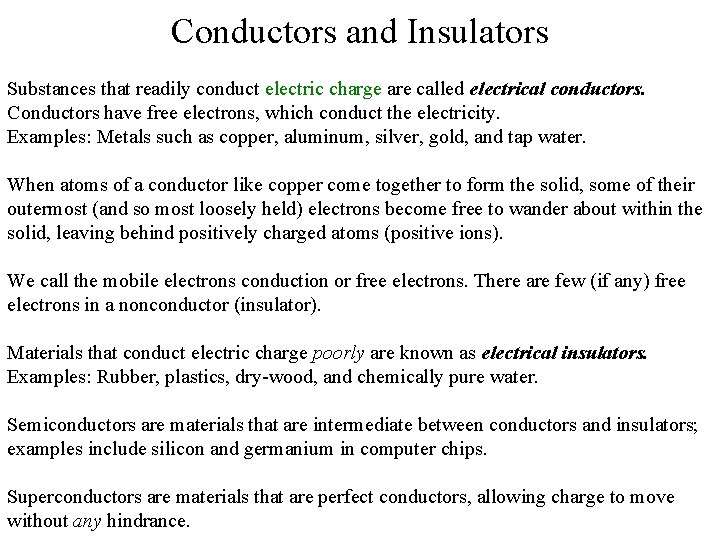Conductors and Insulators Substances that readily conduct electric charge are called electrical conductors. Conductors have free electrons, which conduct the electricity. Examples: Metals such as copper, aluminum, silver, gold, and tap water. When atoms of a conductor like copper come together to form the solid, some of their outermost (and so most loosely held) electrons become free to wander about within the solid, leaving behind positively charged atoms (positive ions). We call the mobile electrons conduction or free electrons. There are few (if any) free electrons in a nonconductor (insulator). Materials that conduct electric charge poorly are known as electrical insulators. Examples: Rubber, plastics, dry-wood, and chemically pure water. Semiconductors are materials that are intermediate between conductors and insulators; examples include silicon and germanium in computer chips. Superconductors are materials that are perfect conductors, allowing charge to move without any hindrance.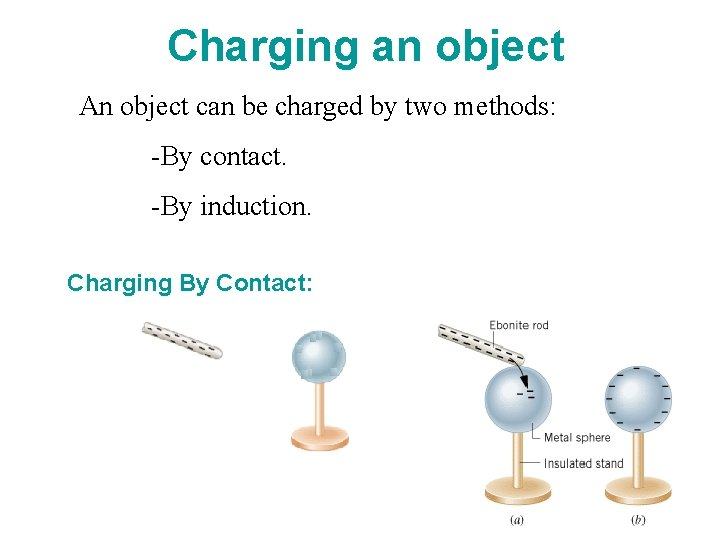Charging an object An object can be charged by two methods: -By contact. -By induction. Charging By Contact:Charging By InductionApplications of electrostatics The Van de Graaff Generator Electrostatic Precipitators and filters Read textbook, Cleaning up the smoke, box 12. 1 (won’t work for sulfur, mercury, and CO 2) Research Paper on Clean Air Copy machines and printersCoulomb's Law Two charged particles attract each other if they have the opposite sign of charge, and repel each other if they have the same signs of charge. This force of repulsion or attraction due to the charge properties of objects is called an electrostatic force. The equation giving the force for charged particles is called Coulomb's law after Charles-Augustin de Coulomb. k = Coulomb’s constant ε 0 = permittivity of free space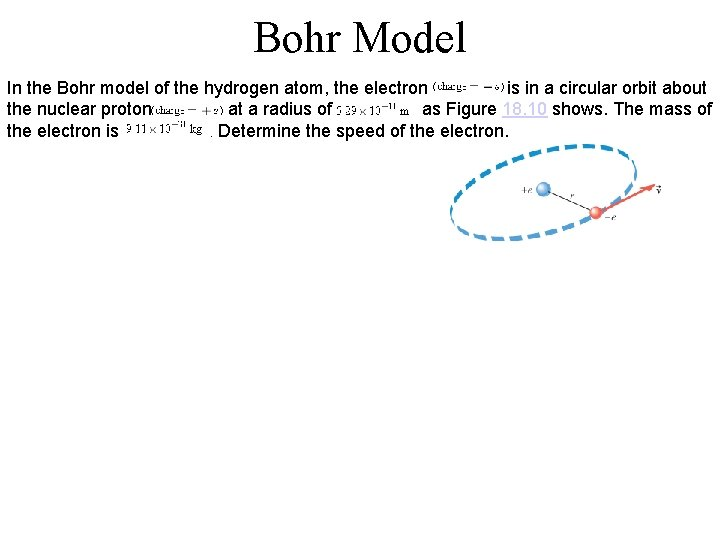Bohr Model In the Bohr model of the hydrogen atom, the electron is in a circular orbit about the nuclear proton at a radius of as Figure 18. 10 shows. The mass of the electron is. Determine the speed of the electron.Ex-4: Three Charges on aline Figure 18. 12 a shows three point charges that lie along the x axis in a vacuum. Determine the magnitude and direction of the net electrostatic force on.The Electric Field The electric field is present in any region of space if there exists electric forces on charges. These electric forces can be detected using a test charge. Test charges are theoretical positive charges that do not alter the electric field to be detected. Electric field at a point in space is defined as the electric force per unit test charge placed at that point. The SI unit for the electric field is the newton per coulomb (N/C). Electric field is a vector.